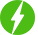# Algorithms in Competitive Programming: Maths Puzzles II(1)

1

Algorithms in Competitive Programming: Maths Puzzles II(1), Covering algorithms used in combinatorial game theory puzzles.

## Course Description

This is a series of courses on popular algorithms used especially in the field of competitive programming!

Competitive programming is a mental sport that tests students on a variety of logical or mathematical problems, and the use of algorithms is essential in improving the efficiency of your solution.

The aim of competitive programming is to write source code of computer programs that are able to solve given problems. Typical such tasks belong to one of the following categories: combinatorics, number theory, graph theory, algorithmic game theory, computational geometry, string analysis, and data structures.

This course focuses not only on the implementation of algorithms but also on the mathematics behind these algorithms since they are requisite in understanding the correctness and efficiency of the algorithms. Specifically, rigid proofs for algorithms will be covered in this series of lectures.

This is the first course in the series Algorithms in Competitive Programming: Maths Puzzles II. In this course, we will be learning about combinatorial game theory, a rather niche topic in competitive programming. However, it is(arguably) one of the most interesting topics and there is more mathematical logic behind these combinatorial puzzles than you would have imagined.

A typical course structure is as follows:

– Problem description

– A naive approach

– Introducing the algorithm

– Mathematical proof of the correctness and efficiency of the algorithm

– A sample problem

– Short quiz

– Hands-on practice

We will be happy to hear your thoughtsRegister New Account
• Total (0)
0